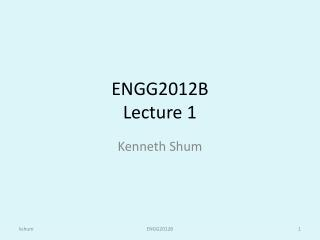DownloadDownload PresentationENGG2012B Lecture 1

# ENGG2012B Lecture 1

Download Presentation## ENGG2012B Lecture 1

- - - - - - - - - - - - - - - - - - - - - - - - - - - E N D - - - - - - - - - - - - - - - - - - - - - - - - - - -
##### Presentation Transcript

1. ENGG2012BLecture 1 Kenneth Shum ENGG2012B

2. Lecturer • SHUM W. K. Kenneth(沈穎祺) • Office: SHB 736 • inside Institute of Network Coding • Email: wkshum @ … • Web: kshum.com • Office hour: T, W 2:00~3:00, or by appointment ENGG2012B

3. Tutors • LONG Feichi (龍非池) • Office: SHB 729 • Email: lf012 @ … • YU Haoran (余皓然) • Office: SHB 825 • Email: yh012 @ … 729 is a power of 3 729 = 333333 ENGG2012B

4. Web • Course webpage https://course.ie.cuhk.edu.hk/~engg2012b/ • E-learning platform https://elearn.cuhk.edu.hk • Announcements, discussions etc. ENGG2012B

5. Syllabus • Complex Variables • complex number, analytic functions, complexfunctions, power series and convergence tests • Linear Algebra • matrices, systems of linear equations and solutions, determinants, rank, eigenvalues and eigenvectors • Numerical Methods • Numerical integration and differentiation, numerical methods in linear algebra • Probability and statistics ENGG2012B

6. Learning Outcome • Manipulate complex numbers and complex functions comfortably • Express a function as a power series • Be familiar with a variety of convergence tests • Determine the type of a matrix (e.g. orthogonal, unitary, Hermitian …) • Solve equations using matrices. • Calculate basic probabilities and statistics ENGG2012B

7. Grading • Biweekly homework, 12% • Midterm test, 38% • Final exam, 50% ENGG2012B

8. Academic Honesty • Attention is drawn to University policy and regulations on honesty in academic work, and to the disciplinary guidelines and procedures applicable to breaches of such policy and regulations. Details may be found at http://www.cuhk.edu.hk/policy/academichonesty/ ENGG2012B

9. Reference texts “Advanced Engineering Mathematics” by Ervin Kreyszig, 10th edition, John Wiley & Sons. “Advanced Engineering Mathematics” by Michael Greenberg, 2nd edition, Prentice Hall. Erwin O. Kreyszig (6/1/1922~12/12/2008) ENGG2012B

10. Course outline • Before midterm (no calculus, more algebraic) • Linear algebra, vector, matrix • Numerical methods for linear algebra • Discrete probability • Complex variables and functions • After midterm (with calculus, more analytic) • Power series • Numerical methods for integration • Continuous probability • Complex differentiation and integration ENGG2012B

12. System of two equations Two variables, two equations ENGG2012B

13. System of three equations Three variables, three equations ENGG2012B

14. System of Linear Equations Multiple variables, multiple equations How to solve it? ENGG2012B

15. Determinant • Area of parallelogram (c,d) (a,b) ENGG2012B

16. 3x3 Determinant • Volume of parallelepiped (g,h,i) (d,e,f) (a,b,c) ENGG2012B

17. Nutrition problem • Find a combination of food A, B, C and D in order to satisfy the nutrition requirement exactly. How to solve it using linear algebra? ENGG2012B

18. Electronic Circuit (static) • Find the current through each resistor System of linear equations ENGG2012B

19. Electronic Circuit (dynamic) • Find the current through each resistor This is a topic in ENGG2011 alternatingcurrent inductor System of differential equations ENGG2012B

20. Electronic Circuit (stochastic) • How about randomness and thermal noise? This is a topic in ELEG3410 thermal noise alternatingcurrent Inductance variesaccording to voice ENGG2012B

21. Probability • What is the chance of winning the first prize of mark six? • Binomial coefficient • The total number of ways that we can pick k out of n distinct object = • Notations: • (pronounced as “n choose k”) http://www.youtube.com/watch?v=ETFOTboBMHE ENGG2012B

22. Expected gain • Probability of first prize • Suppose that the first prize is \$100,000,000, and you have \$10. Decision Not buy buy Expected value \$7.15 \$10 ENGG2012B

23. Complex numbers • Useful in solving polynomials Im Complexplane Re ENGG2012B

24. Roots of unity Complex plane Im -1 Re 1 ENGG2012B